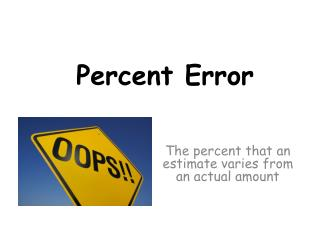DownloadDownload PresentationThe percent that an estimate varies from an actual amount

# The percent that an estimate varies from an actual amount

Télécharger la présentation## The percent that an estimate varies from an actual amount

- - - - - - - - - - - - - - - - - - - - - - - - - - - E N D - - - - - - - - - - - - - - - - - - - - - - - - - - -
##### Presentation Transcript

1. Percent Error The percent that an estimate varies from an actual amount

2. Vocabulary • The percent error is: • a ratio that compares the inaccuracy of an estimate, or the amount of error, to the actual amount. • To find the percent error, the proportion used is:

3. Ex 1) As a fundraiser, the Student Activities Council sold chances to guess the number of jelly beans in a jar. Brenda won the raffle with a guess of 370 jelly beans even though there were actually 400 jelly beans in the jar. What was the percent error? Subtract the guess and the actual value to find the amount of error. The percent error between Brenda’s guess and the actual amount is 7.5%.

4. Ex 2) George is adding a rail around his back porch, and he estimates that the length of the porch is 13.5 feet. The actual length is 15 feet. Find the percent error in George’s estimate. Subtract the estimate and the actual value to find the amount of error. The percent error between George’s estimate and the actual amount is 10%.

5. Ex 3) The odometer in Mr. Washington’s car does not work correctly. The odometer recorded 26.4 miles for his last trip to the hardware store, but he knows the distance traveled is 30 miles. What is the percent error? Subtract the odometer’s mileage from the actual distance to find the amount of error. The percent error between the car’s odometer and the actual distance was 12%.

6. Ex 4) The exact value for the density of aluminum is 2.699 g/cm³. Working in the science lab at school, Joseph finds the density of a piece of aluminum to be 2.75 g/cm³. What is Joseph’s percent error? Round your answer to the nearest hundredth. Subtract Joseph’s calculated density from the actual density find the amount of error. The percent error between Joseph’s findings and aluminum’s actual density was 1.89%.